Question

Let us consider the following three statements:

I. Recursively enumerable languages are those that can be accepted by a Turing machine;

II. Recursive languages are those that can be decided by a Turing machine;

III. A recursively enumerable language accepted by a Turing machine that halts is recursive.

Which of the following holds?

a.Only I;
b.Only II;
c.Only I and II;
d.Only II and III;
e. All I, II, and III.

e. All I, II, and III.

Explanation:

Recursive Enumerable (RE)

RE languages or type-0 languages are generated by type-0 grammars. An RE language can be accepted or recognized by Turing machine which means it will enter into final state for the strings of language and may or may not enter into rejecting state for the strings which are not part of the language. It means TM can loop forever for the strings which are not a part of the language. RE languages are also called as Turing recognizable languages.

A recursively enumerable language is a formal language for which there exists a Turing machine (or other computable function) that will halt and accept when presented with any string in the language as input but may either halt and reject or loop forever when presented with a string not in the language. Contrast this to recursive languages, which require that the Turing machine halts in all cases.

Recursive Language (REC)

A recursive language (subset of RE) can be decided by Turing machine which means it will enter into final state for the strings of language and rejecting state for the strings which are not part of the language. e.g.; L= {anbncn | n>=1} is recursive because we can construct a turing machine which will move to final state if the string is of the form anbncn else move to non-final state. So the TM will always halt in this case. REC languages are also called as Turing decidable languages.

#### Earn Coins

Coins can be redeemed for fabulous gifts.

Similar Homework Help Questions
• ### Question 9. Consider the language {a"b" : n >0}. (i) Is this a regular language? Why...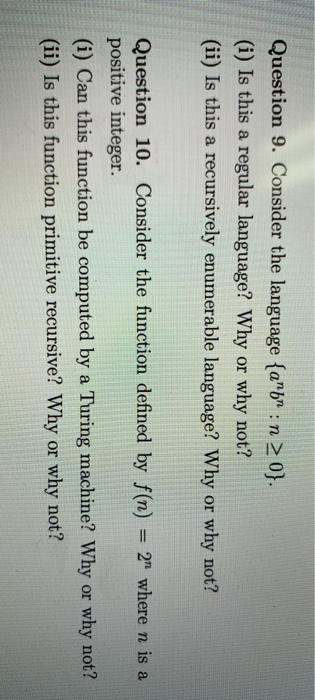Question 9. Consider the language {a"b" : n >0}. (i) Is this a regular language? Why or why not? (ii) Is this a recursively enumerable language? Why or why not? Question 10. Consider the function defined by f(n) = 2 where n is a positive integer. (i) Can this function be computed by a Turing machine? Why or why not? (ii) Is this function primitive recursive? Why or why not?

• ### Determining whether languages are finite, regular, context free, or recursive 1. (Each part is worth 2 points) Fill in the blanks with one of the following (some choices might not be used): a) finit...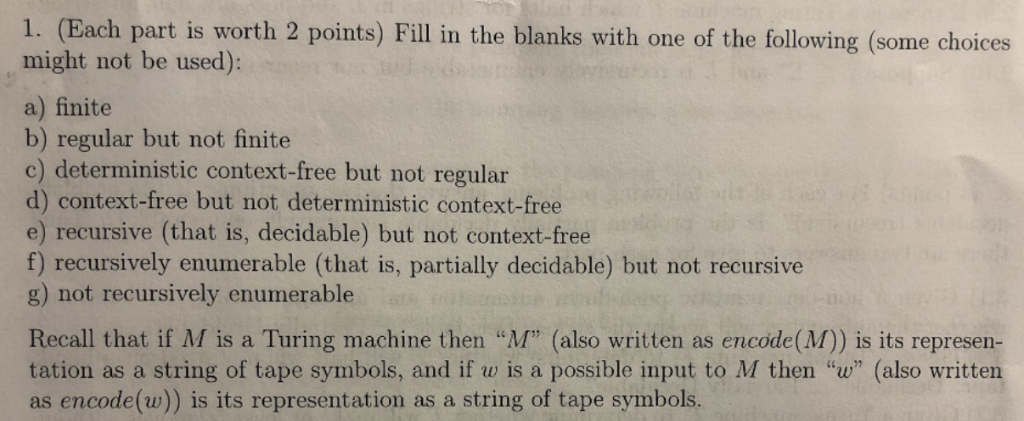Determining whether languages are finite, regular, context free, or recursive 1. (Each part is worth 2 points) Fill in the blanks with one of the following (some choices might not be used): a) finite b) regular but not finite d) context-free but not deterministic context-free e) recursive (that is, decidable) but not context-free f) recursively enumerable (that is, partially decidable) but not recursive g) not recursively enumerable Recall that if M is a Turing machine then "M" (also written as...

• ### If L1 and L2 are Regular Languages, then L1  ∪ L2  is a CFL. Group of answer choices...

If L1 and L2 are Regular Languages, then L1  ∪ L2  is a CFL. Group of answer choices True False Flag this Question Question 61 pts If L1 and L2 are CFLs, then L1  ∩ L2 and L1 ∪ L2 are CFLs. Group of answer choices True False Flag this Question Question 71 pts The regular expression ((ac*)a*)* = ((aa*)c*)*. Group of answer choices True False Flag this Question Question 81 pts Some context free languages are regular. Group of answer choices True...

• ### Consider the function deﬁned by f(n) = 2 nwhere n is a positive integer. (i) Can...

Consider the function deﬁned by f(n) = 2 nwhere n is a positive integer. (i) Can this function be computed by a Turing machine? Why or why not? (ii) Is this function primitive recursive? Why or why not?

• ### Simple java question Consider the following statements related to AtomicInteger's compareAndSet(int expect, int up...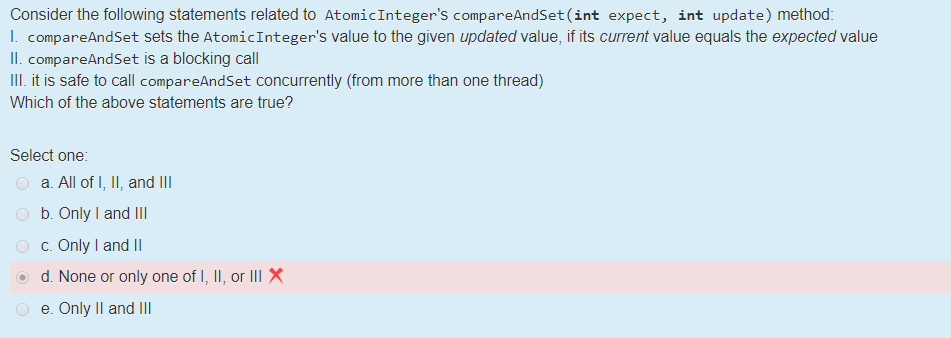Simple java question Consider the following statements related to AtomicInteger's compareAndSet(int expect, int update) method: LcompareAndSet sets the AtomicInteger's value to the given updated value, if its current value equals the expected value II. compareAndSet is a blocking call II. it is safe to call compareAndSet concurrently (from more than one thread) Which of the above statements are true? Select one a. All of I, I, and III b.Only I and III C. Only I and || d. None or...

• ### Consider the following four statements concerning hypothesis tests. I. We can decrease the probability of a...

Consider the following four statements concerning hypothesis tests. I. We can decrease the probability of a Type II error by increasing the sample size. II. We can decrease the probability of a Type II error by decreasing the sample size. III. We can decrease the probability of a Type I error by increasing the level of significance . IV. We can decrease the probability of a Type I error by decreasing the level of significance . Which statements above are...

• ### I need help with that 5. Let Σ-ta, b). Write the δ function for the following...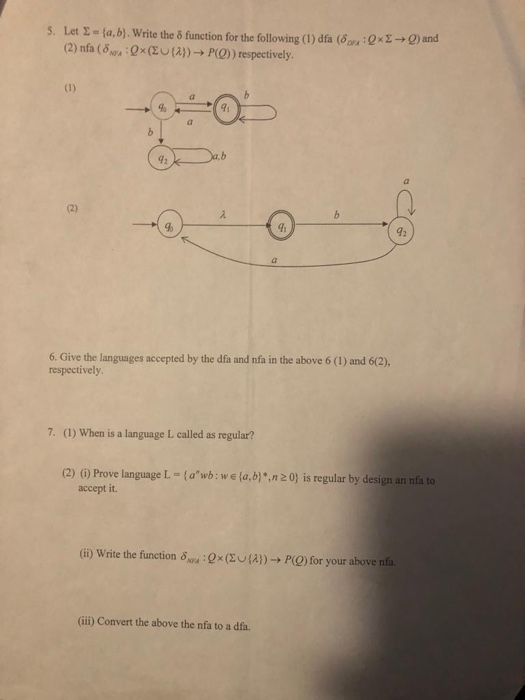I need help with that 5. Let Σ-ta, b). Write the δ function for the following (1) dfa (δου'Qu Σ-Q) and (2) nfa (5,ra : Q x (BU {λ)) → P(D) respectively. 92 92 6. Give the languages accepted by the dfa and nfa in the above 6 (1) and 6(2), respectively 7. (1) When is a language L called as regular? (2) (i) Prove language L = {а"wb: we {a, b) *,n2 O} įs regular by design an nfa...

• ### Consider the following statements concerning a positive integer : (i.) if n is a multiple of...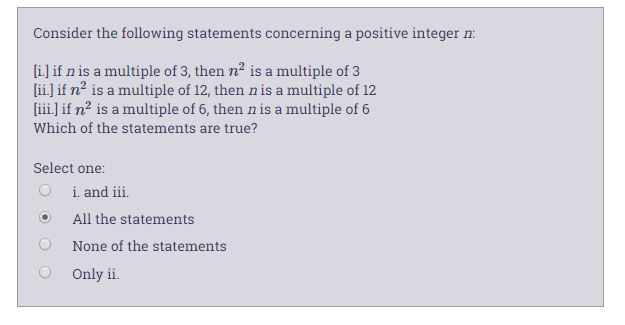Consider the following statements concerning a positive integer : (i.) if n is a multiple of 3, then n2 is a multiple of 3 [ii.) if n' is a multiple of 12, then n is a multiple of 12 (iii.) if nis a multiple of 6, then nis a multiple of 6 Which of the statements are true? Select one: O i. and iii. O All the statements None of the statements Only ii.

• ### 5) Consider the following statements: I: If two triangles are congruent, then they have equal def...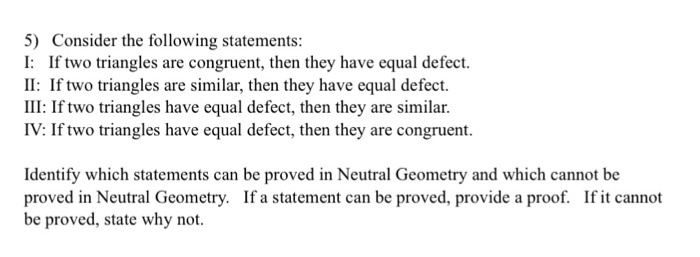5) Consider the following statements: I: If two triangles are congruent, then they have equal defect. II: If two triangles are similar, then they have equal defect. III: If two triangles have equal defect, then they are similar. IV: If two triangles have equal defect, then they are congruent. Identify which statements can be proved in Neutral Geometry and which cannot be proved in Neutral Geometry. If a statement can be proved, provide a proof. If it cannot be proved,...

• ### Which of the following statements describes the processes that can occur during reduction? I. Loss of...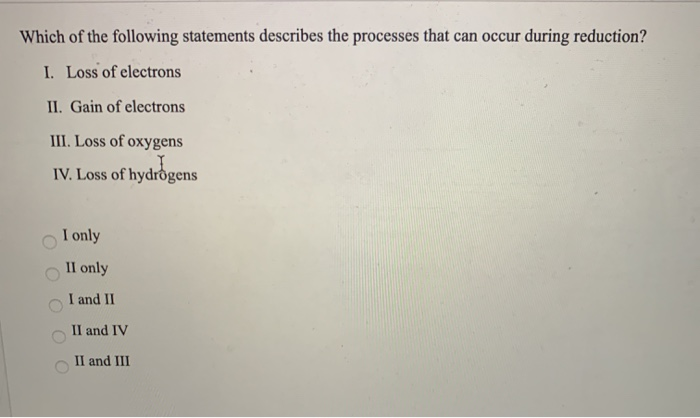Which of the following statements describes the processes that can occur during reduction? I. Loss of electrons II. Gain of electrons III. Loss of oxygens IV. Loss of hydrogens I only II only I and II II and IV II and III